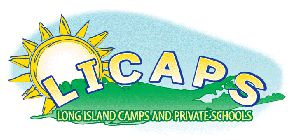# Mathematics

The Middle School mathematics program provides students with opportunities and inclinations to see math as sensible, useful and worthwhile. We encourage our students to view mathematical problem solving and model building as essential, intellectual and creative processes. To ensure uniform success for all, specific tasks are presented at different levels of difficulty as determined through frequent teacher assessment with more accelerated students engaging in higher level mathematical exploration. For students who require a more moderately paced curriculum, mathematical concepts are presented sequentially with additional practice and reinforcement. This more personal approach to math instruction is made possible through small group instruction, individual tutorials and the expertise of teachers who build confidence and “can do” attitudes. Students in all grades are assigned homework to maximize their understanding of math concepts. Additionally, online math resources are used where students can participate in unlimited practice and get immediate feedback or correction. Results reported to the teacher help determine each student’s proficiency.

The fifth grade curriculum consist of concepts of numbers and operations in base 10, algebraic thinking, fractions, measurement systems, interpreting data to solve problems involving information presented in a graph and more. Students develop an in depth understanding of geometry through a kinesthetic approach. Through various inquiry based learning labs, there are extensive interactions with various 2-dimensional and 3-dimensional objects to deconstruct the formulas created that help to calculate area, surface area and volume. Our sixth grade mathematics curriculum utilizes the strong foundations developed from their previous years to explore whole numbers, decimals and fractions, integers, percentages, rations and proportions with depth and rigor to provide students with the confidence and endurance to tackle pre-algebra in 7th grade. Seventh grade mathematics begins with a focus on solving and graphing single step and multi-step algebraic equations. Areas covered include solving number equations and inequalities, graphs and functions, radicals and polynomials, and probability and statistics. Foundations of geometry are reviewed and three dimensional geometric figures and systems of measurement are explored. Students become immersed in abstract thinking involving mathematical concepts. Problem-solving techniques require students to communicate their solutions in writing or orally using the language of mathematics. The eighth grade Algebra I mathematics program extends and applies concepts which were introduced in previous grades. Some math topics studied in eighth grade include rational numbers and radicals, positive and negative exponents, simultaneous linear equations, two and three dimensional geometry, and solving and graphing linear and exponential equations. Students are encouraged and prepared by the teacher to sit for the New York State Algebra I Regents Exam. Upon graduation, students leave on track for honors classes based on their course work at Ivy League.